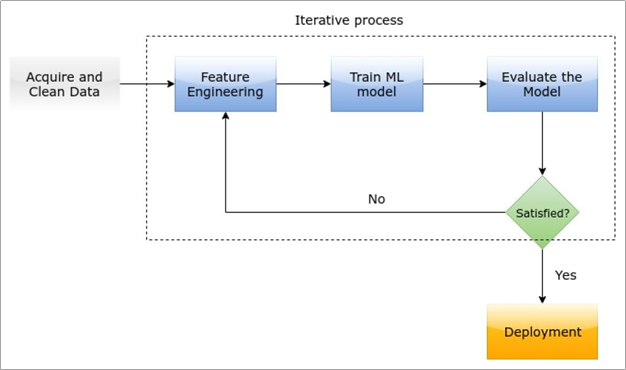Help Center

# Sample Machine Learning problem

Let’s understand ML better by analyzing the weather problem. Assume that you want to play soccer on the weekend. Consider this small data set of various weather conditions.

### Problem statement

Based on the dataset of weather conditions, predict whether you will be able to play soccer on the weekend.

### Features/attributes

Based on the data set, the features (also known as attributes) of this problem are:

• Outlook
• Temperature
• Humidity
• Windy

This information is called ‘feature’ or ‘attribute’ because it helps to determine which weather conditions are favorable for playing soccer on a specific day.

### Target variable/Outcome

Based on the data set, the target variable (also known as outcome) is ‘Play’. You are required to predict what the outcome (also known as target) will be i.e. you must be able to determine whether you will be able to play the game.

### Vector

A vector is a row in the data set. For example, in the data set for this problem Sunny, Hot, High, False, No is a vector. No is the target for this vector.

### ML model

An ML model is a model where an algorithm learns patterns from the given data set (known as training data set) to be able to make predictions on a new data set (known as test data set).

### Evaluation metrics

After a model is trained, the next step is to evaluate the model’s performance i.e. to check how accurately the model has learned from the data. This performance is determined by using mathematical formulas.

## Typical learning process

The process that is used by an ML algorithm to learn from the data is as follows: